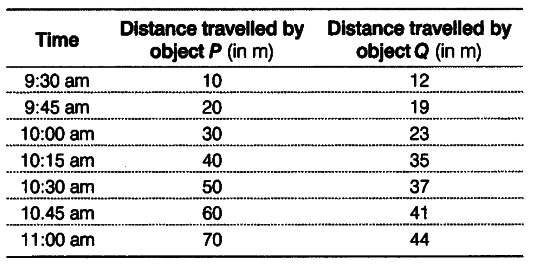# The data regarding the motion of two different objects

The data regarding the motion of two different objects P and Q are given in the following table. Examine . them carefully and state whether the motion of the objects is uniform or non-uniform.We can see that the object P covers a distance of 10 m in every 15 min. In other words, it covers equal distance in equal intervals of time. So, the motion of object P is uniform.On the other hand, the object Q covers 7 m from 9:30 am to 9:45 am, 4 m from 9:45 am to 10:00 am and so on. In other words, it covers unequal distances in equal intervals of time. So, the motion of object Q is non-uniform.